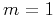Asymptotic pseudounitary stacking operatorsNext: Post-Stack Time Migration Up: Migration Previous: 2. Zero-offset migration

### 3. Common-offset migration

In the case of common-offset migration in a general variable-velocity medium, the weighting function (46) cannot be simplified to a different form, and all its components need to be calculated explicitly by dynamic ray tracing (Cerveny and de Castro, 1993). In the constant-velocity case, we can differentiate the explicit expression for the summation path(50)

whereandare the lengths of the incident and reflected rays:(51)(52)

For simplicity, the vertical component of the midpointis set here to zero. Evaluating the second derivative term in formula (46) for the common-offset geometry leads, after some heavy algebra, to the expression(53)

Substituting (53) into the general formula (46) yields the weighting function for the common-offset true-amplitude constant-velocity migration:(54)

Equation (54) is similar to the result obtained by Sullivan and Cohen (1987). In the case of zero offset, it reduces to equation (49). Note that the value ofin (54) corresponds to the two-dimensional (cylindric) waves recorded on the seismic line. A special case is the 2.5-D inversion, when the waves are assumed to be spherical, while the recording is on a line, and the medium has cylindric symmetry. In this case, the modeling weighting function (42) transforms to (Deregowski and Brown, 1983; Bleistein, 1986)(55)

and the time filter is. Combining this result with formula (53) for, we obtain the weighting function for the 2.5-D common-offset migration in a constant velocity medium (Sullivan and Cohen, 1987):(56)

The corresponding time filter for 2.5-D migration is.

In the common-offset case, the pseudo-unitary weighting is defined from (47) and (53) as follows:(57)

where(58)Asymptotic pseudounitary stacking operatorsNext: Post-Stack Time Migration Up: Migration Previous: 2. Zero-offset migration

2013-03-03